# Selina Solutions Concise Mathematics Class 6 Chapter 14: Fractions Exercise 14(A)

Selina Solutions Concise Mathematics Class 6 Chapter 14: Fractions Exercise 14(A) deals with the study of the conversion of fractions from one type to another. The solutions are solved by BYJU’S experts, as per the latest ICSE exam and help students to obtain competence on the concept covered under this exercise. Various shortcut techniques are available to speed up the problem solving ability among students. Download the Selina Solutions Concise Mathematics Class 6 Chapter 14 Fractions Exercise 14(A) PDF, from the links which are mentioned below.

## Selina Solutions Concise Mathematics Class 6 Chapter 14: Fractions Exercise 14(A) Download PDF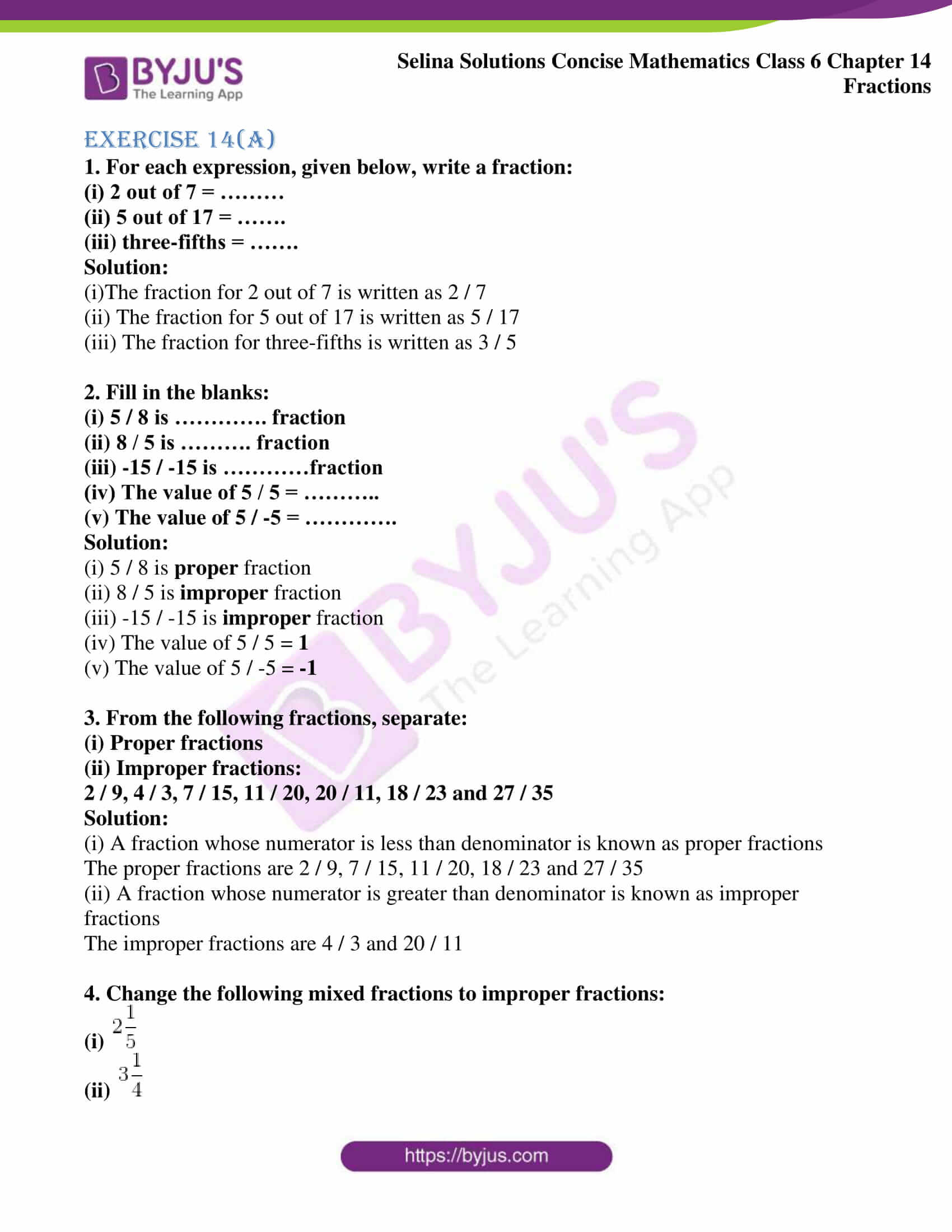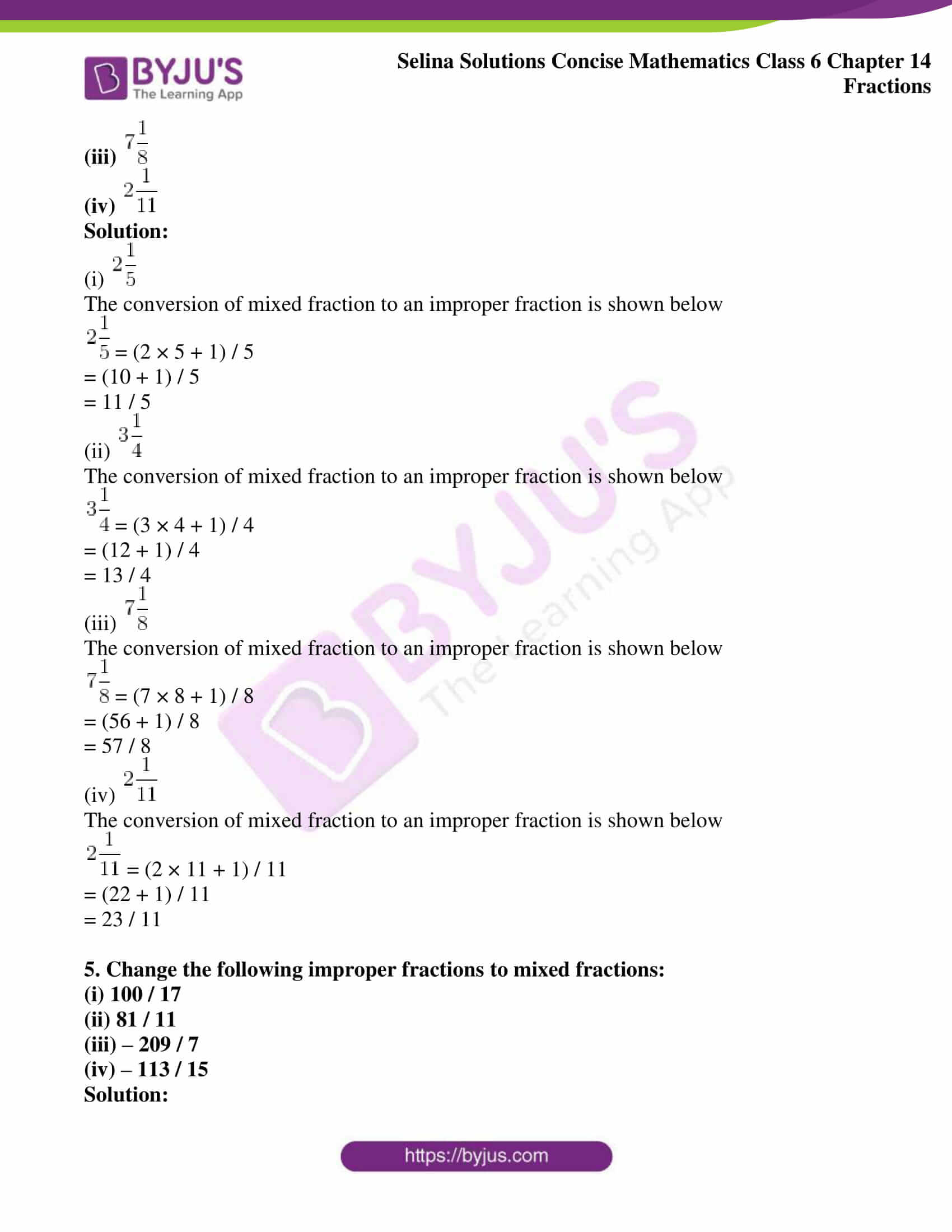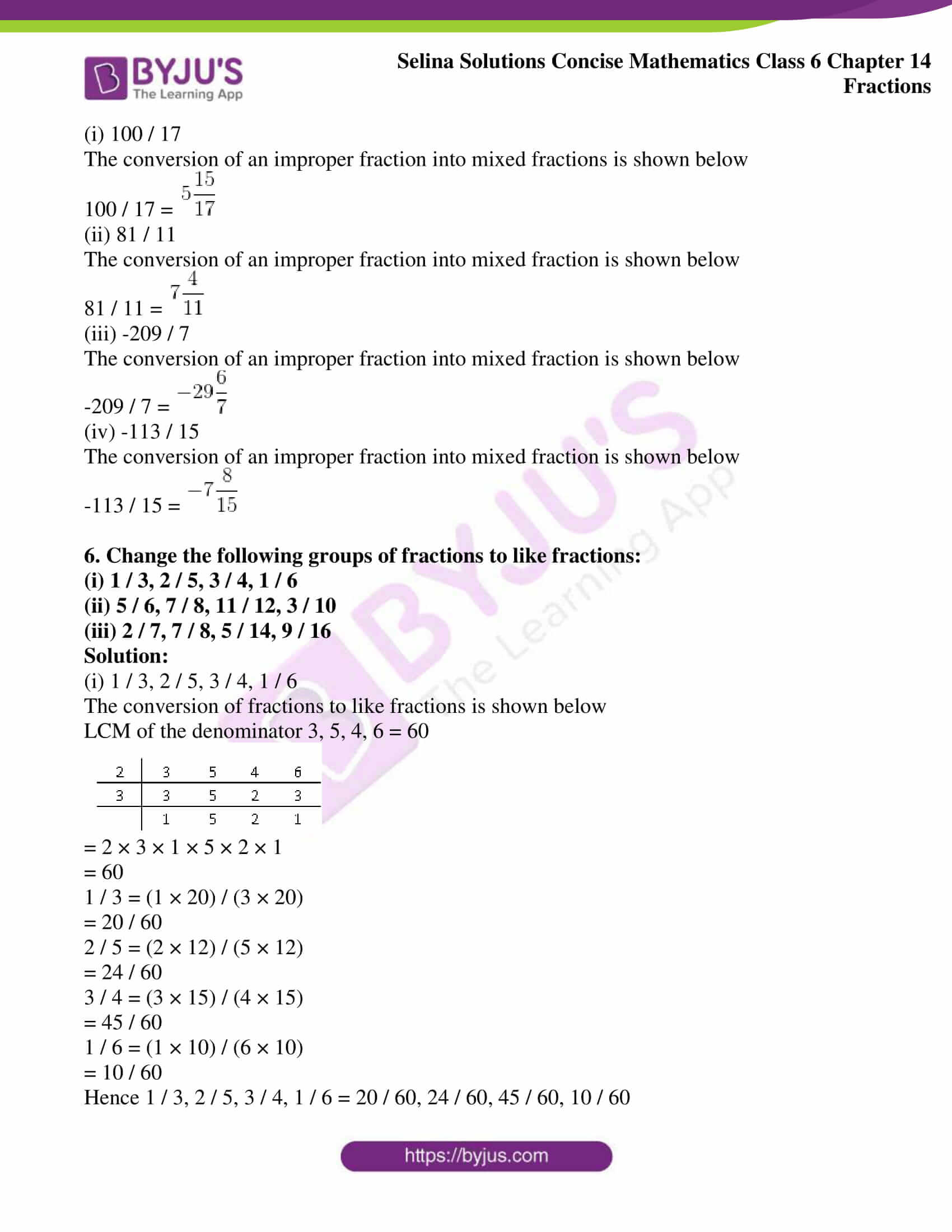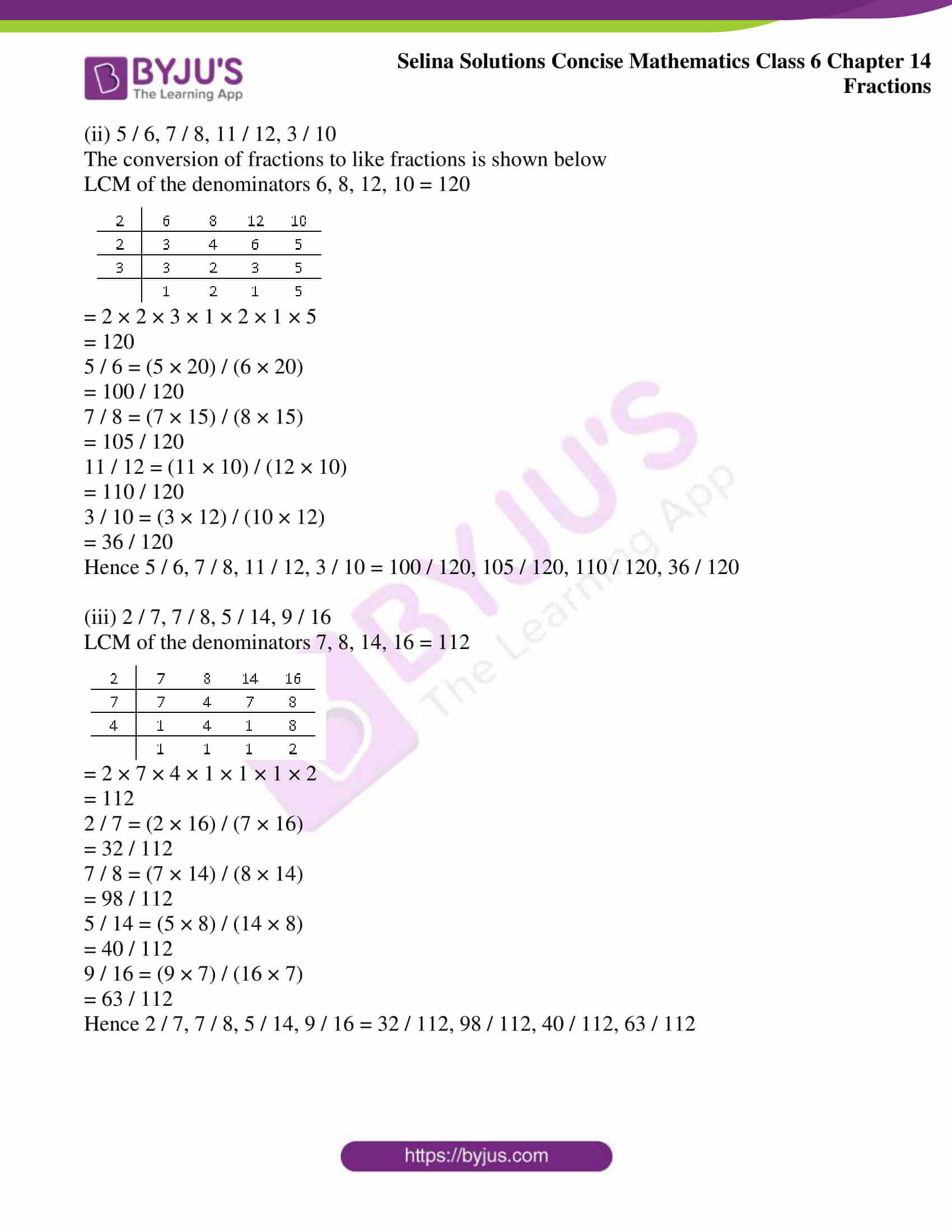### Access other exercises of Selina Solutions Concise Mathematics Class 6 Chapter 14: Fractions

Exercise 14(B) Solutions

Exercise 14(C) Solutions

Exercise 14(D) Solutions

Exercise 14(E) Solutions

### Access Selina Solutions Concise Mathematics Class 6 Chapter 14: Fractions Exercise 14(A)

Exercise 14(A)

1. For each expression, given below, write a fraction:

(i) 2 out of 7 = ………

(ii) 5 out of 17 = …….

(iii) three-fifths = …….

Solution:

(i)The fraction for 2 out of 7 is written as 2 / 7

(ii) The fraction for 5 out of 17 is written as 5 / 17

(iii) The fraction for three-fifths is written as 3 / 5

2. Fill in the blanks:

(i) 5 / 8 is …………. fraction

(ii) 8 / 5 is ………. fraction

(iii) -15 / -15 is …………fraction

(iv) The value of 5 / 5 = ………..

(v) The value of 5 / -5 = ………….

Solution:

(i) 5 / 8 is proper fraction

(ii) 8 / 5 is improper fraction

(iii) -15 / -15 is improper fraction

(iv) The value of 5 / 5 = 1

(v) The value of 5 / -5 = -1

3. From the following fractions, separate:

(i) Proper fractions

(ii) Improper fractions:

2 / 9, 4 / 3, 7 / 15, 11 / 20, 20 / 11, 18 / 23 and 27 / 35

Solution:

(i) A fraction whose numerator is less than denominator is known as proper fractions

The proper fractions are 2 / 9, 7 / 15, 11 / 20, 18 / 23 and 27 / 35

(ii) A fraction whose numerator is greater than denominator is known as improper fractions

The improper fractions are 4 / 3 and 20 / 11

4. Change the following mixed fractions to improper fractions:

(i)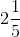(ii)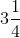(iii)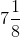(iv)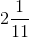Solution:

(i)The conversion of mixed fraction to an improper fraction is shown below= (2 × 5 + 1) / 5

= (10 + 1) / 5

= 11 / 5

(ii)The conversion of mixed fraction to an improper fraction is shown below= (3 × 4 + 1) / 4

= (12 + 1) / 4

= 13 / 4

(iii)The conversion of mixed fraction to an improper fraction is shown below= (7 × 8 + 1) / 8

= (56 + 1) / 8

= 57 / 8

(iv)The conversion of mixed fraction to an improper fraction is shown below= (2 × 11 + 1) / 11

= (22 + 1) / 11

= 23 / 11

5. Change the following improper fractions to mixed fractions:

(i) 100 / 17

(ii) 81 / 11

(iii) – 209 / 7

(iv) – 113 / 15

Solution:

(i) 100 / 17

The conversion of an improper fraction into mixed fractions is shown below

100 / 17 =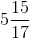(ii) 81 / 11

The conversion of an improper fraction into mixed fraction is shown below

81 / 11 =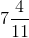(iii) -209 / 7

The conversion of an improper fraction into mixed fraction is shown below

-209 / 7 =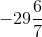(iv) -113 / 15

The conversion of an improper fraction into mixed fraction is shown below

-113 / 15 =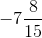6. Change the following groups of fractions to like fractions:

(i) 1 / 3, 2 / 5, 3 / 4, 1 / 6

(ii) 5 / 6, 7 / 8, 11 / 12, 3 / 10

(iii) 2 / 7, 7 / 8, 5 / 14, 9 / 16

Solution:

(i) 1 / 3, 2 / 5, 3 / 4, 1 / 6

The conversion of fractions to like fractions is shown below

LCM of the denominator 3, 5, 4, 6 = 60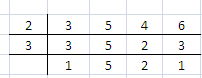= 2 × 3 × 1 × 5 × 2 × 1

= 60

1 / 3 = (1 × 20) / (3 × 20)

= 20 / 60

2 / 5 = (2 × 12) / (5 × 12)

= 24 / 60

3 / 4 = (3 × 15) / (4 × 15)

= 45 / 60

1 / 6 = (1 × 10) / (6 × 10)

= 10 / 60

Hence 1 / 3, 2 / 5, 3 / 4, 1 / 6 = 20 / 60, 24 / 60, 45 / 60, 10 / 60

(ii) 5 / 6, 7 / 8, 11 / 12, 3 / 10

The conversion of fractions to like fractions is shown below

LCM of the denominators 6, 8, 12, 10 = 120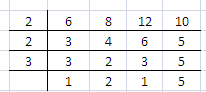= 2 × 2 × 3 × 1 × 2 × 1 × 5

= 120

5 / 6 = (5 × 20) / (6 × 20)

= 100 / 120

7 / 8 = (7 × 15) / (8 × 15)

= 105 / 120

11 / 12 = (11 × 10) / (12 × 10)

= 110 / 120

3 / 10 = (3 × 12) / (10 × 12)

= 36 / 120

Hence 5 / 6, 7 / 8, 11 / 12, 3 / 10 = 100 / 120, 105 / 120, 110 / 120, 36 / 120

(iii) 2 / 7, 7 / 8, 5 / 14, 9 / 16

LCM of the denominators 7, 8, 14, 16 = 112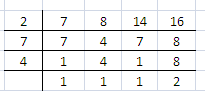= 2 × 7 × 4 × 1 × 1 × 1 × 2

= 112

2 / 7 = (2 × 16) / (7 × 16)

= 32 / 112

7 / 8 = (7 × 14) / (8 × 14)

= 98 / 112

5 / 14 = (5 × 8) / (14 × 8)

= 40 / 112

9 / 16 = (9 × 7) / (16 × 7)

= 63 / 112

Hence 2 / 7, 7 / 8, 5 / 14, 9 / 16 = 32 / 112, 98 / 112, 40 / 112, 63 / 112